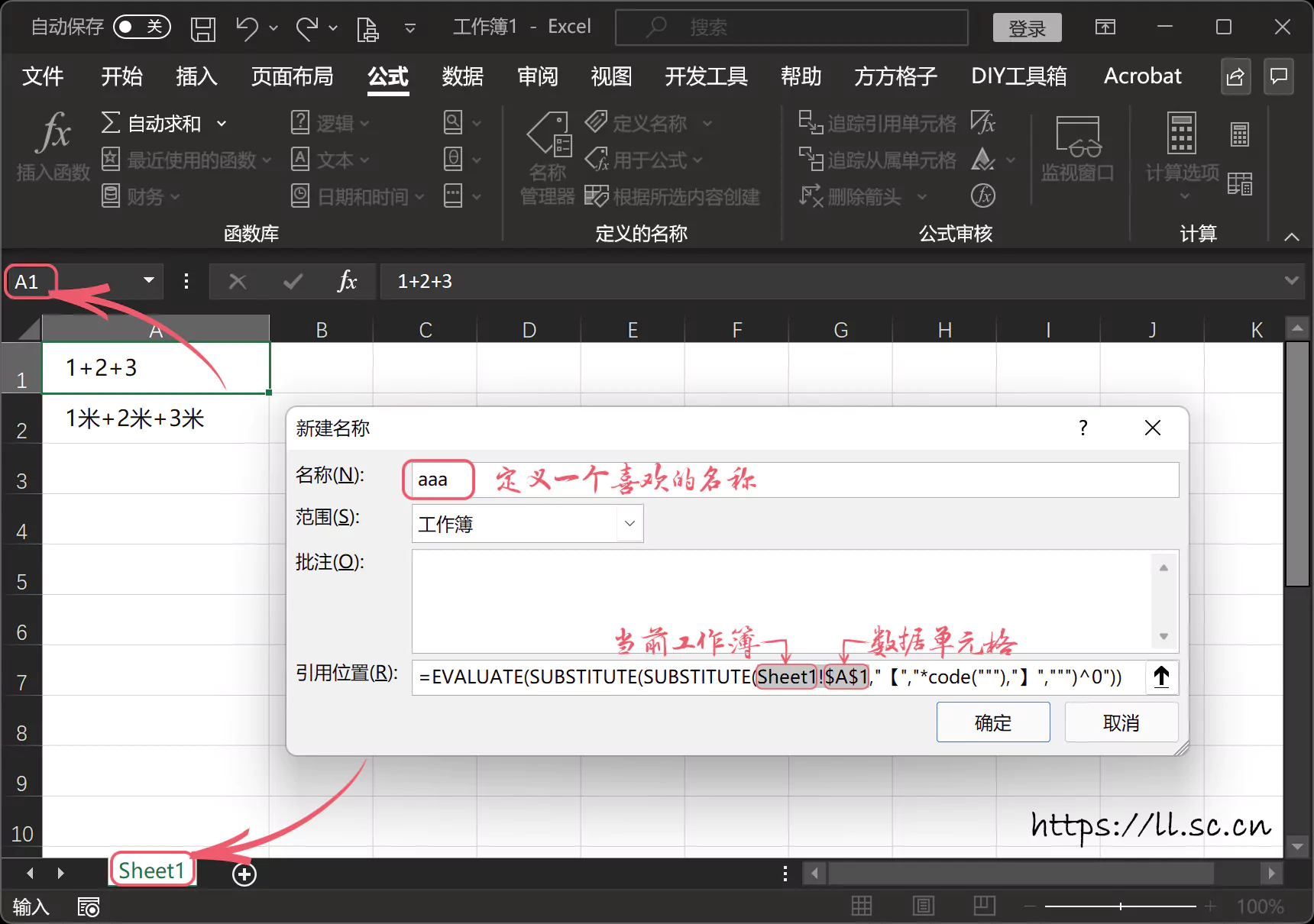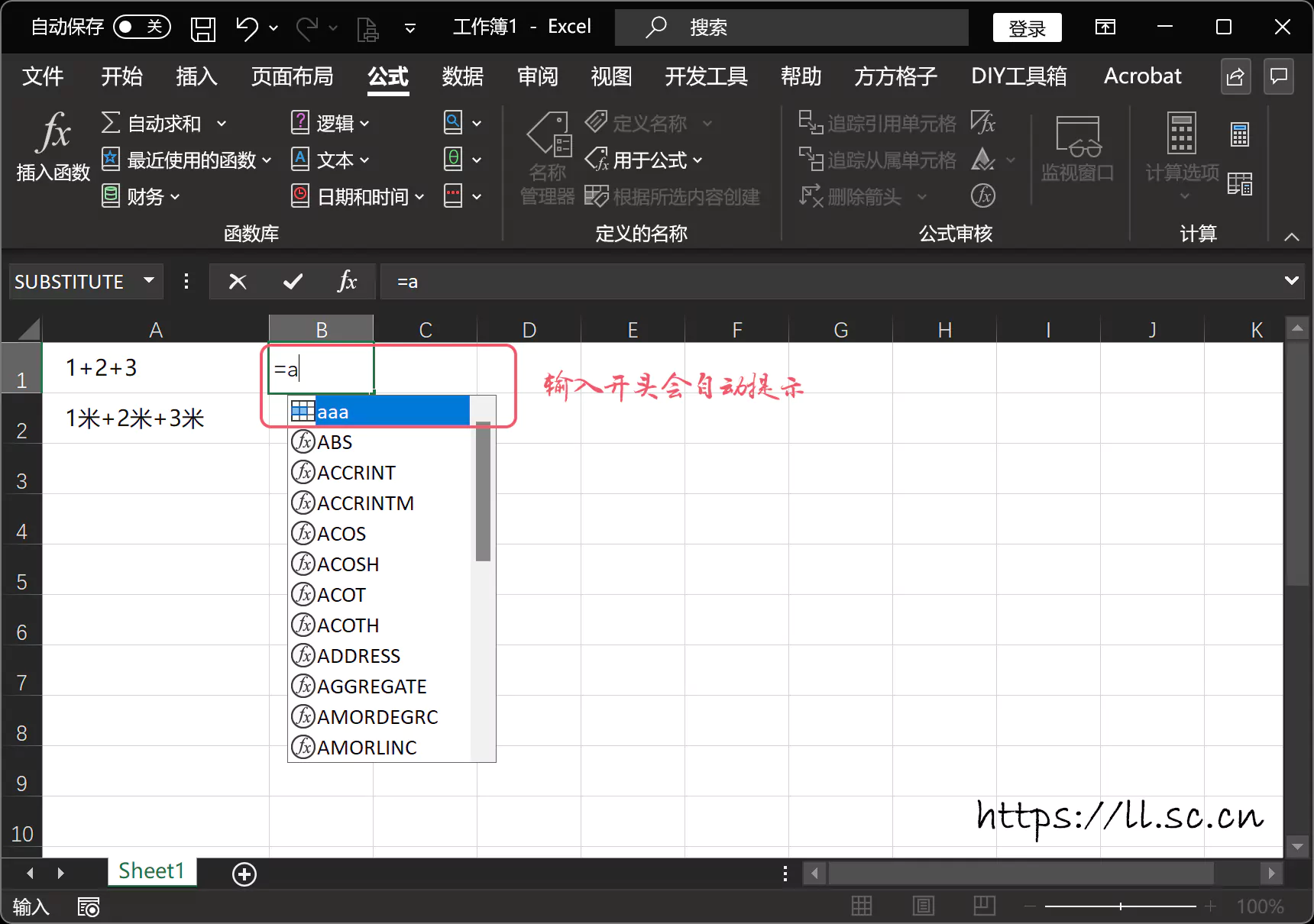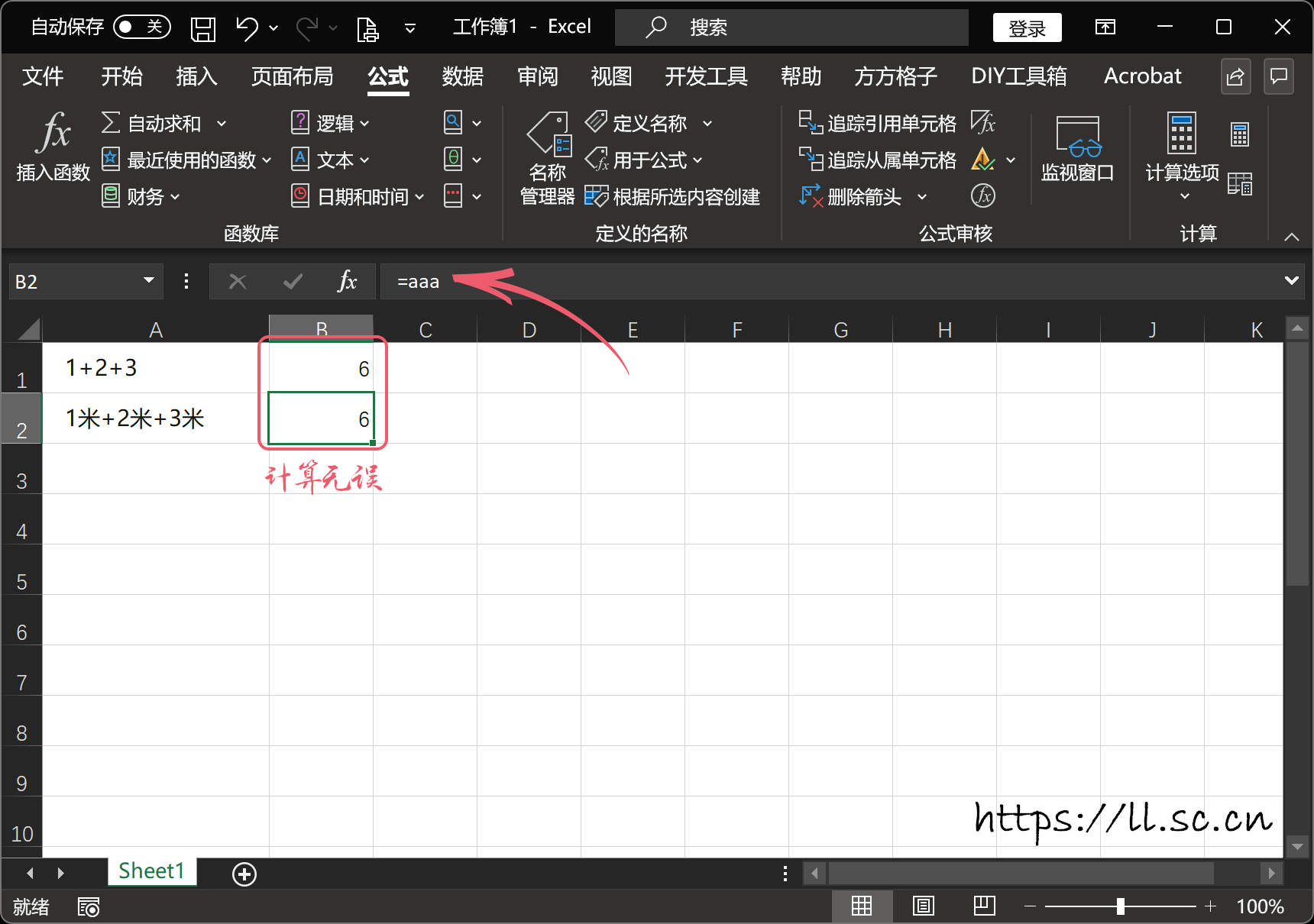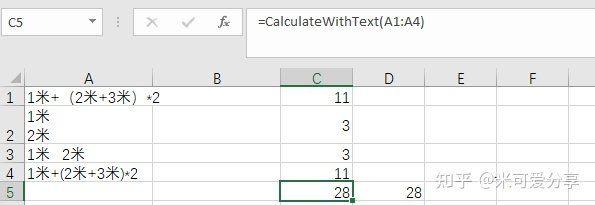# Excel - 包含文字的单元格计算

Excel 的单元格中如果包含中文字符，则计算的时候会出错，接下来我们将解决此问题……

## 解决方法

1 xxx 1+2+3
2 xxx 1米+2米+3米
3 xxx 。。。

### 初级版▲ 新建名称

 1  =EVALUATE(SUBSTITUTE(SUBSTITUTE(Sheet1!$A$1,"【","*code("""),"】",""")^0"))▲ 输入公式▲ 输入定义的名称▲ 计算结果

### 高阶版

#### 解题思路：

1. 去除掉公式中非数字、加减乘除运算符号；
2. 通过 VBA 中的 Evaluate 对最终的公式进行计算；

#### 操作方法

  1 2 3 4 5 6 7 8 9 10 11 12 13 14 15 16 17 18 19 20 21 22 23 24 25 26 27 28 29 30 31 32 33 34 35 36 37 38 39 40 41 42 43 44 45 46 47 48 49 50  'Excel中对带有文本的单元格进行计算 Function CalculateWithText(ParamArray args() As Variant) Dim objFormula As String Dim result As String Dim current As String Dim eachRange As Range For Each Rng In args For Each eachRange In Rng If result <> "" Then result = result + "+" End If result = result + CStr(CalculateText(eachRange.Value)) Next result = CStr(Evaluate(result)) Next Debug.Print result CalculateWithText = Evaluate(result) End Function Function CalculateText(objFormula As String) Dim current As String Dim validSymbol As String validSymbol = "+,-,*,/,.,(,)" '替换换行符、空格等 objFormula = Replace(objFormula, vbNewLine, "+") objFormula = Replace(objFormula, vbCr, "+") objFormula = Replace(objFormula, vbLf, "+") objFormula = Replace(objFormula, vbCrLf, "+") objFormula = Replace(objFormula, " ", "+") '替换中文（、） objFormula = Replace(objFormula, "（", "(") objFormula = Replace(objFormula, "）", ")") For n = 1 To Len(objFormula) current = Mid(objFormula, n, 1) If IsNumeric(current) Or IsInArray(current, Split(validSymbol, ",")) Then result = result + current End If Next n 'Fix当单元格为空时Error 2015，为空设置结果为0 If result <> "" Then CalculateText = Evaluate(result) Else CalculateText = 0 End If End Function Function IsInArray(stringToBeFound As String, arr As Variant) As Boolean IsInArray = (UBound(Filter(arr, stringToBeFound)) > -1) End Function 

• 计算带文本公式
• 支持多区间和单元格计算
• 支持单元格中内容包含换行
• 支持单元格中内容包含空格
• 解决因单元格过多超过公式的最大长度导致结果出现 #VALUE! 的错误
• 解决单元格为空时，出现 #VALUE! 的错误
• 解决 () 未优先计算的问题▲ 计算成功

## 参考链接